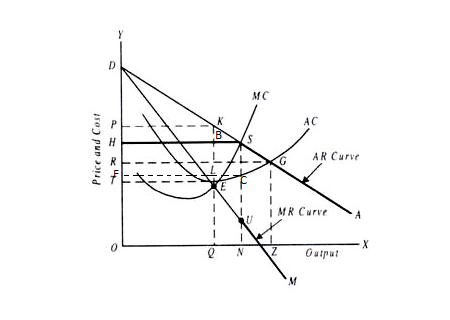# True or false: If a price ceiling is imposed in a natural monopoly market and the price ceiling...

## Question:

True or false: If a price ceiling is imposed in a natural monopoly market and the price ceiling is above marginal cost and average cost of the monopolist, and below the price the monopolist would choose to charge if unregulated, then the profit maximizing monopolist will increase it its production when the price ceiling is imposed.

## Natural Monopoly:

A monopoly is the market structure wherein there is only one seller, with no close substitute, and there are large numbers of buyers.A natural monopoly is a kind of monopoly which is created and has high entry barriers because of very high set up cost of conducting the business.

## Answer and Explanation:

"If a price ceiling is imposed in a natural monopoly market and the price ceiling is above marginal cost and average cost of the monopolist, and below the price the monopolist would choose to charge if unregulated, then the profit maximizing monopolist will increase it its production when the price ceiling is imposed."

• TRUE.

Let's understand this through a graph. Consider the following graph -H is the price ceiling whereas P is the profit maximizing price. If the market is unregulated, the monopolist will sell OQ output. Now, if H is the price that the monopolist can charge, at maximum, and he would still sell OQ, then the profit will be OQBH-OTEQ = HTEB.

Let's assume the monopolist increases the output, at this ceiling price. Now, he sells ON at price OH. At this output and price,the profit will be ONSH-ONCF = FCSH.

Now, notice that the area of FCHS seems more than HTEB. Thus, it will profitable for the monopolist, if he increases the output.

#### Learn more about this topic:Natural Monopoly in Economics: Definition & Examples

from Intro to Business: Help and Review

Chapter 3 / Lesson 13
80K